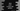# Java program to print a cross star pattern## Java program to print a cross star pattern:

In this post, we will learn how to print a cross star pattern in Java. It will take the value of height of the pattern and it will print the pattern using star or *.

Before we move to the program, let’s learn to write down the algorithm.

### Algorithm to print a cross star pattern:

Suppose we are printing the below cross star pattern:

``````*     *
*   *
* *
*
* *
*   *
*     *``````

Let’s replace the blank spaces with #:

``````*#####*
#*###*#
##*#*##
###*###
##*#*##
#*###*#
*#####*``````

This is the same algorithm. We need to replace the # characters with blank space.

Suppose, i represents the current row and j represents the current column.

• For i = 1, * are printed at j = 1 and j = 7
• For i = 2, * are printed at j = 2 and j = 6
• For i = 3, * are printed at j = 3 and j = 5
• For i = 4, * are printed at j = 4
• For i = 5, * are printed at j = 5 and j = 3 etc.

As you can see here, * are printed if: - i and j are equal - i + j is equal to height + 1, height is 7 here

So, instead of printing #, we have to print a blank space.

``````import java.util.Scanner;

class Main {
public static void main(String[] args) {
Scanner sc = new Scanner(System.in);
int height;

System.out.println("Enter the height: ");
height = sc.nextInt();

for (int i = 1; i < height + 1; i++) {
for (int j = 1; j < height + 1; j++) {
if (i == j || i + j == height + 1) {
System.out.print("*");
} else {
System.out.print(" ");
}
}
System.out.println();
}
}
}``````

It will print output as like below:

``````Enter the height:
7
*     *
*   *
* *
*
* *
*   *
*     *

Enter the height:
9
*       *
*     *
*   *
* *
*
* *
*   *
*     *
*       *``````

Note that, for even value of height, it will print different types of patterns i.e. both lines will not cross at the center:

``````Enter the height:
10
*        *
*      *
*    *
*  *
**
**
*  *
*    *
*      *
*        *``````